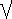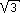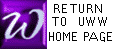(self test)

A quadratic equation is an equation which can be written in the form ax2 + bx + c = 0 where a (≠ 0), b, and c are constants (as opposed to x, which is a variable). To solve an equation such as ax2 + bx + c = 0 by factoring, or by completing the square, check out these links.

For quadratic equations which are difficult to factor, the Quadratic Formula will always reveal solutions. Traditionally, these solutions are written:

x =
- b ±b2 - 4ac2a
Here is an example:

Original Equation: 2x2 + 5x = 25
Re-written equation: 2x2 + 5x - 25 = 0
Identify values of "a", "b", and "c": a = 2, b = 5, c = -25
Substiture these values into the quadratic formula, to get:
x =
-(5) ±(5)2 - 4(2)(-25)2(2)
=   - 5 ± 15
4
=-5 52In other examples, b2 - 4ac might be negative; in this case, x may be imaginary or complex (as in  below).

  Solve using the quadratic formula (see example above). Express real number answers as a decimal accurate to within 0.01, such as "1.33" Express imaginary answers as a+bi and a-bi without spaces, such as "1+3i": x2 + 3x - 9 = 0 x =     or     x =

  Solve using the quadratic formula (see example above). Express real number answers as a decimal accurate to within 0.01, such as "1.33" Express imaginary answers as a+bi and a-bi without spaces, such as "1+3i": x2 + 2x + 2 = 0 x =     or     x =

  Solve using the quadratic formula (see example above). Express real number answers as a decimal accurate to within 0.01, such as "1.33" Express imaginary answers as a+bi and a-bi without spaces, such as "1+3i": x2 + x = 3 x =     or     x =

  Solve using the quadratic formula (see example above). Express real number answers as a decimal accurate to within 0.01, such as "1.33" Express imaginary answers as a+bi and a-bi without spaces, such as "1+3i": x2 - 5x + 5 = 0 x =     or     x =

  Solve using the quadratic formula (see example above). Express real number answers as a decimal accurate to within 0.01, such as "1.33" Express imaginary answers as a+bi and a-bi without spaces, such as "1+3i": x(x + 6) + 13 = 0 x =     or     x =

  Solve using the quadratic formula (see example above). Express real number answers as a decimal accurate to within 0.01, such as "1.33" Express imaginary answers as a+bi and a-bi without spaces, such as "1+3i":x2 + 2x += 0 Then x = or x =

 Solve using the quadratic formula (Methods of section 5.2 may help here).
Express real number answers as a decimal accurate to within 0.01, such as "1.33"
Express imaginary answers as a+bi and a-bi without spaces, such as "1+3i":

 If x+2x+3 - 2x =  3 Then x = or x =

 Solve using the quadratic formula (Methods of section 5.2 may help here).
Express real number answers as a decimal accurate to within 0.01, such as "1.33"
Express imaginary answers as a+bi and a-bi without spaces, such as "1+3i":

 If x+3x-6 - x x-3 = 1 Then x = or x =

  Solve using the quadratic formula (see example above). Express real number answers as a decimal accurate to within 0.01, such as "1.33" Express imaginary answers as a+bi and a-bi without spaces, such as "1+3i": x2 + 1 = 0 Then x = or x =

  Solve using the quadratic formula (see example above). Express real number answers as a decimal accurate to within 0.01, such as "1.33" Express imaginary answers as a+bi or a-bi without spaces, such as "1+3i": x2 + 2ix - 5 = 0 Then x = or x =

 Return toMath 141Home Page